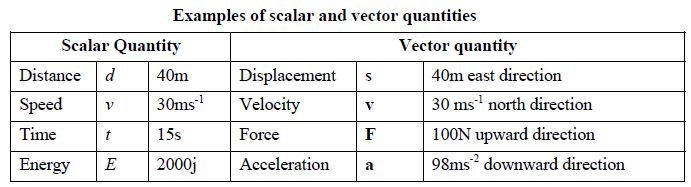Physics

# Explain Scalar and Vector Quantity

Anything that can be measured in this physical world is called a physical quantity. When a physical quantity is measured, it has got a magnitude. We express this magnitude in terms of a number and a unit. For example, if we say that the height of the bench is 1.5 meter, we mean that the unit of length is meter and height of the bench is 1.5 times this unit. But all physical quantities cannot be completely expressed by its magnitude only. To express not the only magnitude of some quantities is needed but also the direction.

For example, if we say that a car is running at the speed of 40 km per hour, it is understood that the car moves a distance 40 km in one hour, but the direction of motion of the car cannot be known from this statement. To know the actual position of the car, the direction of the motion should also be stated. So, we can see, to express some of the quantities completely direction is needed with the magnitude of the quantities. In consideration of direction all quantities of the world we can divide into two categories. Such as

1. In-directional quantity or Scalar quantity
2. Directional quantity or Vector quantity

Scalar quantity: Physical quantities which can be fully expressed by magnitude only are called scalar quantities. Length, mass, speed, work, energy, time, temperature etc are the examples of scalar quantities.

Vector quantity: The physical quantity which needs both magnitude and direction to be fully expressed are called vector quantities. Displacement, velocity, acceleration, force, electric intensity etc are the examples of vector quantities.

Table Shows that vectors are expressed by magnitude and direction whereas scalars are expressed by magnitude only.